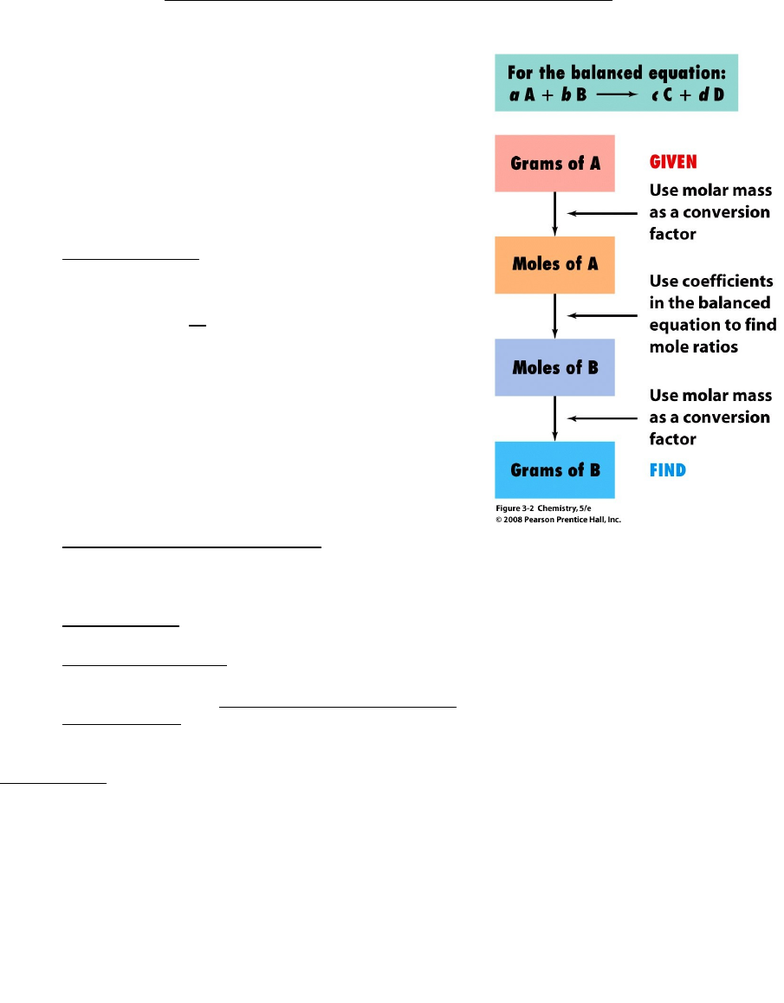Study Guides (390,000)
CA (150,000)
UOIT (500)
CHEM (10)
ifa (3)

# CHEM 1010U Study Guide - Acetic Anhydride, Molar Mass, Chemical Formula

This preview shows pages 1-3. to view the full 12 pages of the document.Chemistry 1010 Chapter 3: Mass Relationships in Chemical Reactions Thursday, Sept 11/14
Balancing Chemical Equations:
Law of mass conservation: mass is neither created nor destroyed in chemical reactions
That means – in a balanced chemical equation:
The kinds of atoms on each side of the equation are the same
The number of atoms on each side of the equations are the same.
1. Write the unbalanced equation using the correct chemical formula for each reactant and
product
2. Find suitable coefficients – the numbers placed before formulas to indicate how many
formula units of each substance are required to balance the equation.
3. Reduce the coefficients to their smallest whole-number values, if necessary, by dividing
them all by a common divisor.
4. Check your answer by making sure that the numbers and kinds of atoms are the same on
both sides of the equation.
What do balanced reactions tell us?
how many moles of each reactant will be needed
mass of each reactant needed
moles of product will be produced (theoretically)
expected mass for the product
Stoichiometry: Chemical Arithmetic
Required for mole-mass conversions
aA+bB => cC+dD
1. Determining molar mass
HCl
H=1.0079 g/mol
Cl=35.453g/mol
=36.431gmol

Only pages 1-3 are available for preview. Some parts have been intentionally blurred.Chemistry 1010 Chapter 3: Mass Relationships in Chemical Reactions Thursday, Sept 11/14
2. Converting from atoms to mole and mole to mass
How many moles of sucrose (C12H22O11) are in a tablespoon of sugar containing
2.85g?
How many molecules of sucrose are in a mole?
2.85g C12H22O11 x 1mol C12H22O11/molar mass(g)= 2.85 g?
2NaOH(aq) + Cl2(g)
NaOCl(aq) + NaCl(aq) + H2O(l)
Ex:
How many grams of NaOH are needed to react with 25.0 g Cl2?
Cl2=35.453 g/mol
+35.453g/mol
=70.906g
Na =22.989 g/mol
O=16.g/mol
H=1.008g/mol
=40.0 g
25.0g Cl2 x 1 mol Cl2 /70.9 g x 2mols of NaOH/1 mol Cl2 x 40.0 g / 1 mol NaOH
=28.2 g NaOH are needed to react with 25.0 g Cl2
C7H6O3 + C4H6O3
C9H8O4 +CH3CO2H
Ex2 :
How many grams of acetic anhydride are needed to react with 4.50 g of salicylic acid?
4.50g C7H6O3 X 1 mol C7H6O3 /138.13 g C7H6O3 X 1 mol C4H6O3/1 mol C7H6O3 X 102.1 g
C4H6O3/ 1 mol C4H6O3 = 3.33 C4H6O3
138.13 =C7H6O3

Only pages 1-3 are available for preview. Some parts have been intentionally blurred.Chemistry 1010 Chapter 3: Mass Relationships in Chemical Reactions Thursday, Sept 11/14
102.1= C4 H6O3
2. How many grams of aspirin (C9H8O4) will result?
4.5 g C7H6O3 X 1 mol C7H6O3 /138.13 g C7H6O3 X 1 mol
C9H8O4/1 mol C7H6O3 x 180.18 C7H6O3 / 1 mol C7H6O3 =
5.87 g
Stoichiometry:
Determining the mass of each reactant
needed or predicting the mass of product
formed are all done in terms of moles and
then converted to masses
It is often necessary to convert back and
forth between mass and moles in any
given problem
Yields of Chemical Reactions
Actual Yield : The amount actually formed in a reaction
Theoretical Yield : The amount predicted by calculations
Percent Yield =
Actual yield of product
Theoretical yield of product x100
Example 1: What is the percent yield of the reaction if 32.8 g of methyl tert-butyl ether is
obtained from reaction of 26.3 g of isobutylene with sufficient methanol?
C4H8
(
g
)
+C H4O(l)
C5H12 O(l)
Methyl tert-butyl ether
(MTBE)
Molar mass = 88.0 g/mol
Isobutylene
Molar mass
= 56.0 g/mol